nhstplot is a fairly simple package to use. This vignette is intended to explain the basics (plotting using the defaults), before showing how to use the options.

# The basics

After installing the library with install.packages("nhstplot") you need to load the library:

library(nhstplot)

‘nhstplot’ is composed of 4 functions, one for each major NHST test “family” :

• $$\chi^2$$ tests (with the plotchisqtest function)
• $$F$$ tests (with the plotftest function)
• $$t$$ tests (with the plotttest function)
• $$z$$ tests (with the plotztest function)

Let’s see how to use each one without changing the graphical options.

## $$\chi^2$$ tests

The plotchisqtest function only requires 2 arguments : The first one is the $$\chi^2$$ value (parameter : chisq), and the second one is the degrees of freedom (parameter df).

Here’s an example with respectively 8 and 4.

plotchisqtest(chisq = 8, df = 4)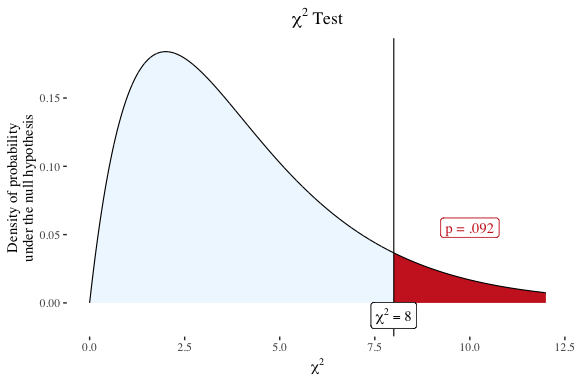Note that the same is achieved with plotchisqtest(8,4).

You can also use the function by passing an object created by chisq.test()

test <- chisq.test(c(A = 37, B = 18, C = 25))
plotchisqtest(test)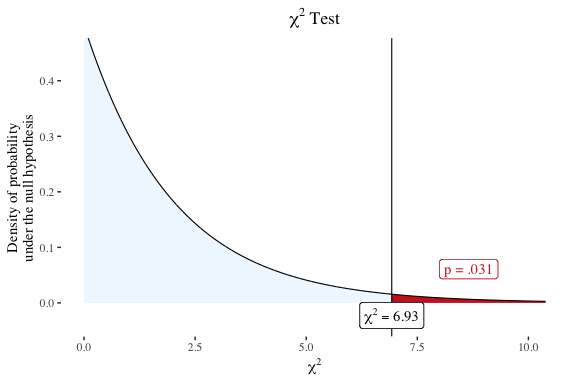## $$F$$ tests

The plotftest function only requires 3 arguments : The first one is the $$F$$ value (parameter : f), and the second and third ones are respectively the degrees of freedom of the numerator (parameter dfnum) and the denominator (parameter dfdenom).

Here’s an example with respectively 4, 3 and 5.

plotftest(f = 4, dfnum = 3, dfdenom = 5)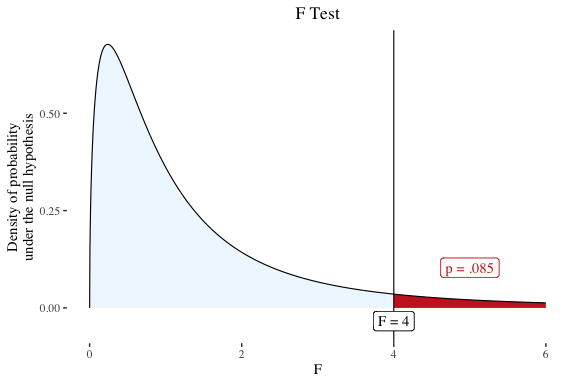Note that the same is achieved with plotftest(4,3,5).

You can also use the function by passing an object created by lm()

x <- rnorm(10) ; y <- x + rnorm(10)
fit <- lm(y ~ x)
plotftest(fit)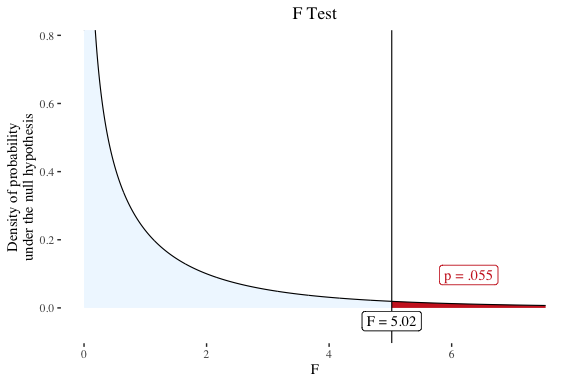## $$t$$ tests

The plotttest function only requires 2 arguments : The first one is the $$t$$ value (parameter : t), and the second one is the degrees of freedom of the numerator (argument df).

Here’s an example with respectively 2 and 10.

plotttest(t = 2, df = 10)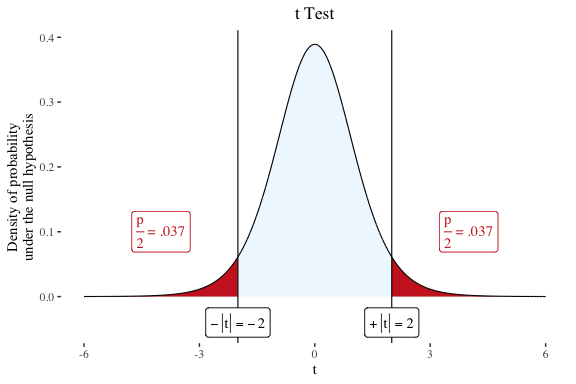Note that the same is achieved with plotttest(2,10).

By default, the plotttest function plots a two-tailed test. However, a one-tailed test can be plotted by adding the argument tails = "one":

plotttest(2, 10, tails = "one")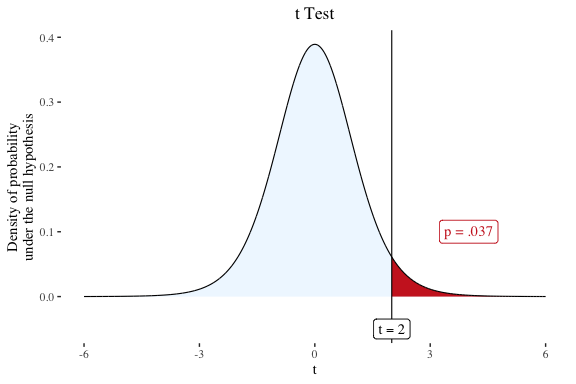The left or right tail is automatically selected using the sign of provided $$t$$:

plotttest(-2, 10, tails = "one")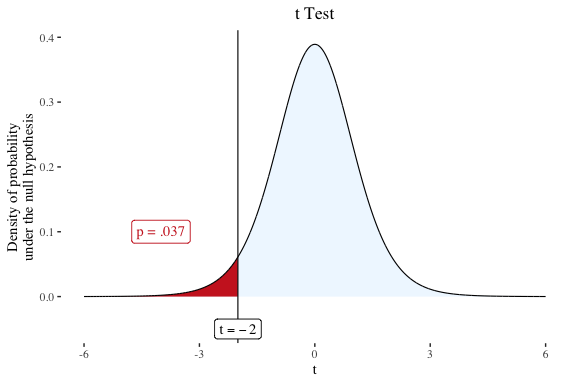You can also use the function by passing an object created by t.test()

test <- t.test(rnorm(10), rnorm(10))
plotttest(test)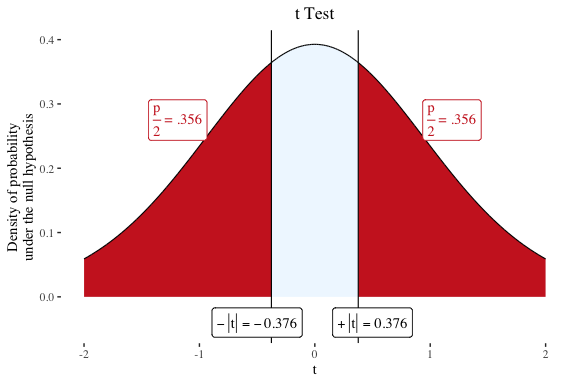…or cor.test().

test <- cor.test(rnorm(10), rnorm(10))
plotttest(test)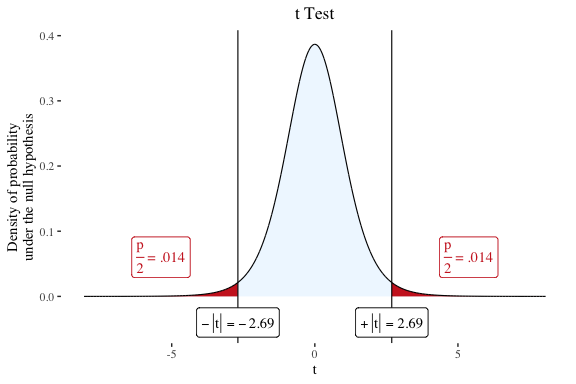## $$z$$ tests

The plotztest function only requires 1 argument : The $$z$$ value (parameter z).

Here’s an example with a $$z$$ value of 2.

plotztest(z = 2)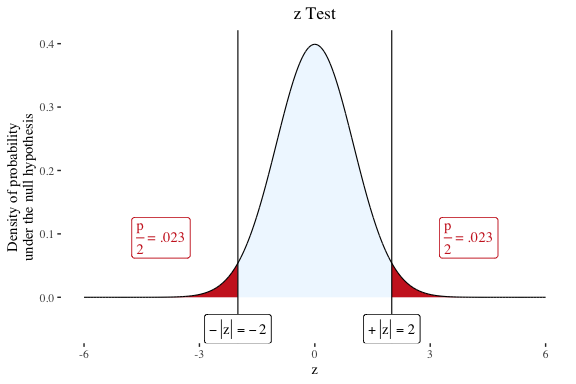Note that the same is achieved with plozttest(2).

By default, the plotztest function plots a two-tailed test. However, a one-tailed test can be plotted by adding the argument tails = "one":

plotztest(2, tails = "one")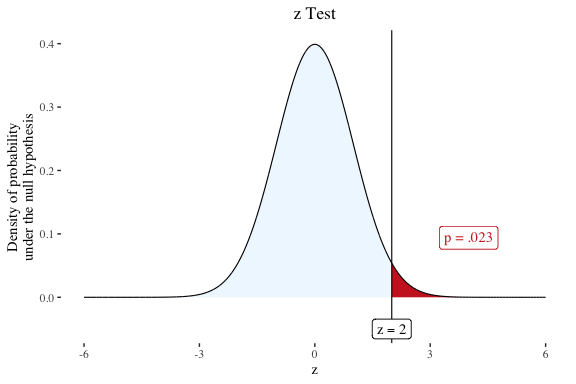The left or right tail is automatically selected using the sign of provided $$t$$:

plotztest(-2, tails = "one")